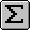# Calculator

The StatsDirect calculator can be used both within StatsDirect and independently as a replacement for the Windows calculator. The calculator evaluates expressions in the form of simple arithmetic or more complex algebra.

All calculations are performed in IEEE-754 double precision (64-bit).

The 'save' button copies the expression currently evaluated and its result to a list from which you can select saved expressions to paste into new ones.

When you close the calculator it will paste saved expressions and results into a report in StatsDirect if the "save results to report on exit" box at the bottom left of the calculator is checked.Constants PI 3.14159265358979323846 (p) EE 2.71828182845904523536 (e) Arithmetic Functions ABS absolute value CLOG common (base 10) logarithm CEXP anti log (base 10) EXP anti log (base e) LOG natural (base e, Napieran) logarithm LOGIT logit: log(p/(1-p), p=proportion ALOGIT antilogit: exp(l)/1+exp(l), l=logit SQR or SQRT square root ! factorial (maximum 170.569) LOG! log factorial IZ normal deviate for a p value UZ upper tail p for a normal deviate LZ lower tail p for a normal deviate TRUNC or FIX integer part of a real number CINT real number rounded to nearest integer INT real number truncated to integer closest to zero

Please note that the largest factorial allowed is 170.569398315538748, but you can work with Log factorials via the LOG! function, e.g. LOG!(272).

 Arithmetic Operators ^ exponentiation (to the power of) + addition - subtraction * multiplication / division \ integer division

Calculations give an order of priority to arithmetic operators, this must be considered when entering expressions. For example, the result of the expression "6 - 3/2" is 4.5 and not 1.5 because division takes priority over subtraction.

Priority of arithmetic operators in descending order

1. Exponentiation (^)
2. Negation (-X) (Exception = x^-y; i.e. 4^-2 is 0.0625 and not -16)
3. Multiplication and Division (*, /)
4. Integer Division (\)
5. Addition and Subtraction (+, -)

 Trigonometric Functions ARCCOS arc cosine ARCCOSH arc hyperbolic cosine ARCCOT arc cotangent ARCCOTH arc hyperbolic cotangent ARCCSC arc cosecant ARCCSCH arc hyperbolic cosecant ARCTANH arc hyperbolic tangent ARCSEC arc secant ARCSECH arc hyperbolic secant ARCSIN arc sine ARCSINH arc hyperbolic sine ATN arc tangent COS cosine COT cotangent COTH hyperbolic cotangent CSC cosecant CSCH hyperbolic cosecant SIN sine SINH hyperbolic sine SECH hyperbolic secant SEC secant TAN tangent TANH hyperbolic tangent

To convert degrees to radians, multiply degrees by pi/180. To convert radians to degrees, multiply radians by 180/pi.

 Logical Functions AND logical AND NOT logical NOT OR logical OR < less than = equal to > greater than